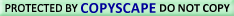HUNGRY? TRY comCustom Search

Hey, you're in the power supply zone--YOUR HANDYMAN ZONE!

Power Supply 1-2-3: Direct Current (DC)

# DC NETWORK ANALYSIS

### Mesh current by inspection

We take a second look at the �mesh current method� with all the currents runing counterclockwise (ccw). The motivation is to simplify the writing of mesh equations by ignoring the resistor voltage drop polarity. Though, we must pay attention to the polarity of voltage sources with respect to assumed current direction. The sign of the resistor voltage drops will follow a fixed pattern.

If we write a set of conventional mesh current equations for the circuit below, where we do pay attention to the signs of the voltage drop across the resistors, we may rearrange the coefficients into a fixed pattern:Once rearranged, we may write equations by inspection. The signs of the coefficients follow a fixed pattern in the pair above, or the set of three in the rules below.

• Mesh current rules:
• This method assumes electron flow (not conventional current flow) voltage sources. Replace any current source in parallel with a resistor with an equivalent voltage source in series with an equivalent resistance.
• Ignoring current direction or voltage polarity on resistors, draw counterclockwise current loops traversing all components. Avoid nested loops.
• Write voltage-law equations in terms of unknown currents currents: I1, I2, and I3. Equaton 1 coefficient 1, equation 2, coefficient 2, and equation 3 coefficient 3 are the positive sums of resistors around the respective loops.
• All other coefficients are negative, representative of the resistance common to a pair of loops. Equation 1 coefficent 2 is the resistor common to loops 1 and 2, coefficient 3 the resistor common to loops 1 an 3. Repeat for other equations and coefficients.
`+(sum of R's loop 1)I1 - (common R loop 1-2)I2 - (common R loop 1-3)I3   = E1`
`-(common R loop 1-2)I1 + (sum of R's loop 2)I2 - (common R loop 2-3)I3   = E2`
`-(common R loop 1-3)I1 - (common R loop 2-3)I2 + (sum of R's loop 3)I3   = E3`
• The right hand side of the equations is equal to any electron current flow voltage source. A voltage rise with respect to the counterclockwise assumed current is positive, and 0 for no voltage source.

• Solve equations for mesh currents:I1, I2, and I3 . Solve for currents through individual resistors with KCL. Solve for voltages with Ohms Law and KVL.

While the above rules are specific for a three mesh circuit, the rules may be extended to smaller or larger meshes. The figure below illustrates the application of the rules. The three currents are all drawn in the same direction, counterclockwise. One KVL equation is written for each of the three loops. Note that there is no polarity drawn on the resistors. We do not need it to determine the signs of the coefficients. Though we do need to pay attention to the polarity of the voltage source with respect to current direction. The I3counterclockwise current traverses the 24V source from (+) to (-). This is a voltage rise for electron current flow. Therefore, the third equation right hand side is +24V.In Octave, enter the coefficients into the A matrix with column elements comma separated, and rows semicolon separated. Enter the voltages into the column vector b. Solve for the unknown currents: I1, I2, and I3 with the command: x=A\b. These currents are contained within the x column vector. The positive values indicate that the three mesh currents all flow in the assumed counterclockwise direction.

`           octave:2> A=[300,-100,-150;-100,650,-300;-150,-300,450]`
`           A =`
`             300  -100  -150`
`             -100  650  -300`
`             -150  -300  450`
` `
`           octave:3> b=[0;0;24]`
`           b =`
`              0`
`              0`
`             24`
` `
`           octave:4> x=A\b`
`           x =`
`             0.093793`
`             0.077241`
`             0.136092`

The mesh currents match the previous solution by a different mesh current method.. The calculation of resistor voltages and currents will be identical to the previous solution. No need to repeat here.

Note that electrical engineering texts are based on conventional current flow. The loop-current, mesh-current method in those text will run the assumed mesh currents clockwise.[aef] The conventional current flows out the (+) terminal of the battery through the circuit, returning to the (-) terminal. A conventional current voltage rise corresponds to tracing the assumed current from (-) to (+) through any voltage sources.

One more example of a previous circuit follows. The resistance around loop 1 is 6 Ω, around loop 2: 3 Ω. The resistance common to both loops is 2 Ω. Note the coefficients of I1 and I2 in the pair of equations. Tracing the assumed counterclockwise loop 1 current through B1 from (+) to (-) corresponds to an electron current flow voltage rise. Thus, the sign of the 28 V is positive. The loop 2 counter clockwise assumed current traces (-) to (+) through B2, a voltage drop. Thus, the sign of B2 is negative, -7 in the 2nd mesh equation. Once again, there are no polarity markings on the resistors. Nor do they figure into the equations.The currents I1 = 5 A, and I2 = 1 A are both positive. They both flow in the direction of the counterclockwise loops. This compares with previous results.

• Summary:
• The modified mesh-current method avoids having to determine the signs of the equation coefficients by drawing all mesh currents counterclockwise for electron current flow.
• However, we do need to determine the sign of any voltage sources in the loop. The voltage source is positive if the assumed ccw current flows with the battery (source). The sign is negative if the assumed ccw current flows against the battery.
• See rules above for details.Use of/Visit to this site constitutes the User's Understanding of and Consent to  | Privacy Policy All links found on this site should be considered as Ad links.  See Ad Disclosures for more details.Monitored for Copyright Compliance